# Linear Regression

## Build a Model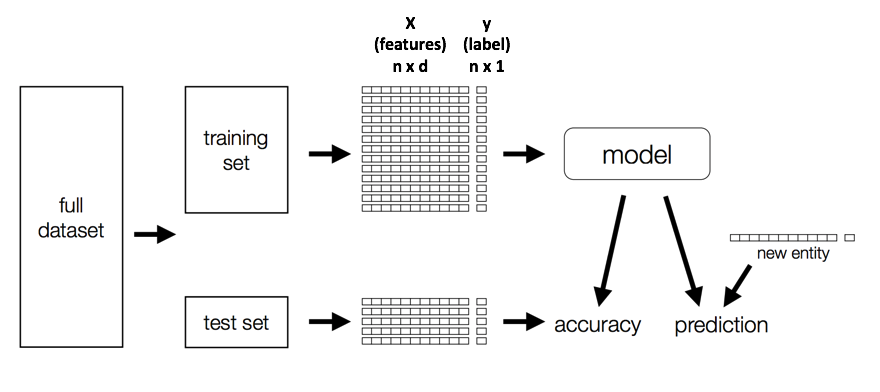## Temporal Data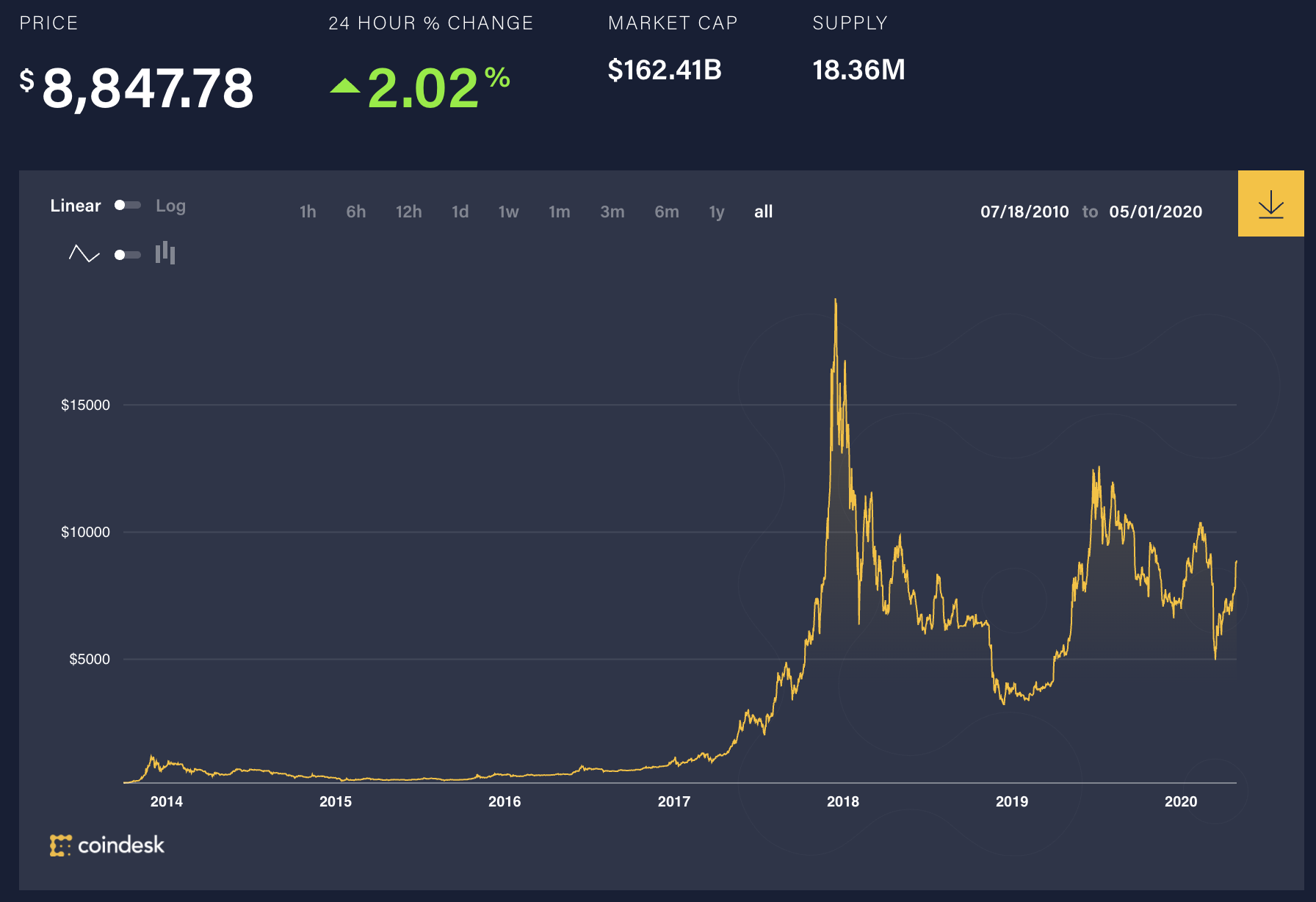## Linear Regression

Goal: Find line of best fit

$\hat{y} = w_{0} + w_{1}x$

y $\approx \hat{y} + \epsilon$

x: feature

y: label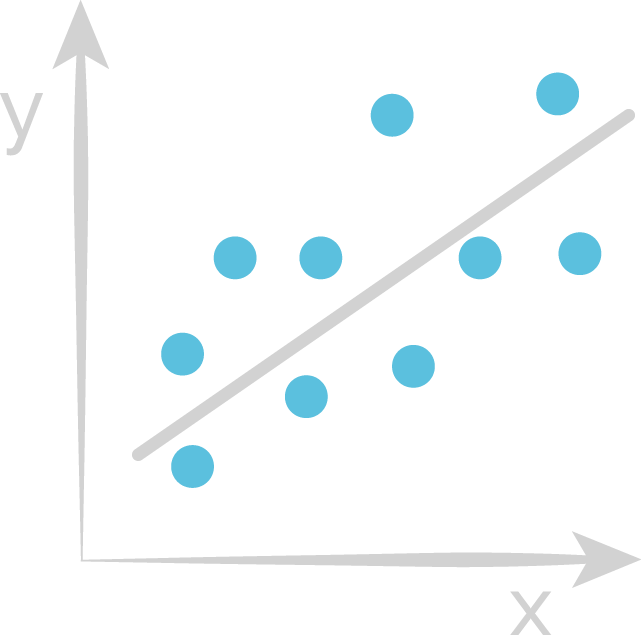#### Learn weights that minimize the residuals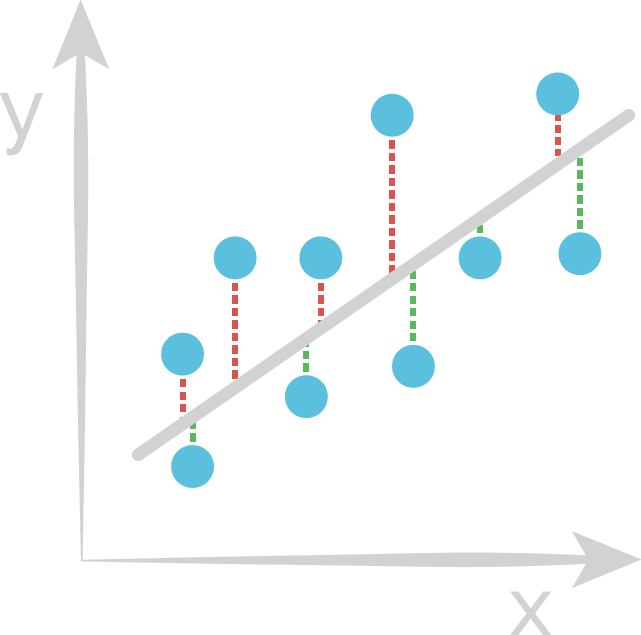• Blue point: True value
• Red line: Positive residual
• Green line: Negative residual

## Regression Evaluator

Measure "closeness" between label and prediction

Evaluation metrics:

• Loss: $(y - \hat{y})$
• Absolute loss: $|y - \hat{y}|$
• Squared loss: $(y - \hat{y})^2$

## Evaluation metric: RMSE

$Error = (y_{i} - \hat{y_{i}})$

$SE = (y_{i} - \hat{y_{i}})^2$

$SSE = \sum_{i=1}^n (y_{i} - \hat{y_{i}})^2$

$MSE = \frac{1}{n}\sum_{i=1}^n (y_{i} - \hat{y_{i}})^2$

$RMSE = \sqrt{\frac{1}{n}\sum_{i=1}^n (y_{i} - \hat{y_{i}})^2}$

## Train vs. Test RMSE

Which is more important? Why?

## R2

Another measurement of "goodness of fit"

$SS_{\text{tot}}=\sum_{i=1}^n(y_{i}-{\bar {y}})^{2}$

$SS_{\text{res}}=\sum_{i=1}^n(y_{i}-\hat{y_{i}})^{2}$

$R^{2} = 1-{SS_{\rm {res}} \over SS_{\rm {tot}}}$

What is the range of R2?

## Machine Learning Libraries

Can train multiple scikit-learn models in parallel with Joblib or Pandas UDFs, but what if our data or model get big...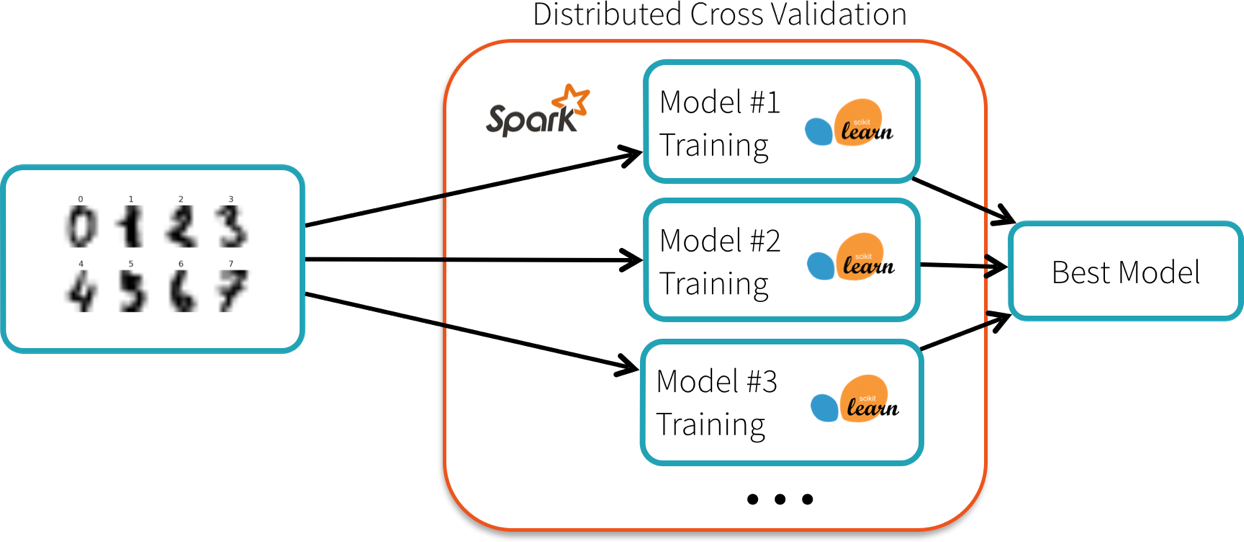## ML Libraries in Spark

### MLlib

Original Spark ML API based on RDDs

### SparkML

Spark 2.0: Entered maintenance mode

Supported API moving forward

## How to Handle Non-Numeric Features?

Categorical

• No intrinsic ordering
• e.g. Gender, Country, Occupation

Ordinal

• Relative ordering, but inconsistent spacing between categories
• e.g. Excellent, good, poor

## One idea

Create single numerical feature to represent non-numeric one

Categorical features:

• Animal = {'Dog', 'Cat', 'Fish'}
• 'Dog' = 1, 'Cat' = 2, 'Fish' = 3

Implies Cats are 2x dogs!

## One Hot Encoding (OHE)

Create a ‘dummy’ feature for each category

'Dog' => [1, 0, 0], 'Cat' => [0, 1, 0], 'Fish' => [0, 0, 1]

No spurious relationships!

## Storage Space

Ok, so that works if we only have a few animal types, but what if we had a zoo?

## Sparse Vectors

Size of vector, indices of non-zero elements, values


DenseVector(0, 0, 0, 7, 0, 2, 0, 0, 0, 0)
SparseVector(10, [3, 5], [7, 2])


## Assumptions?

Linear relationship between X and Y

Features not correlated

Errors only in Y##### Which ones are suited to Linear Regression?Slide credit G. Calmattes